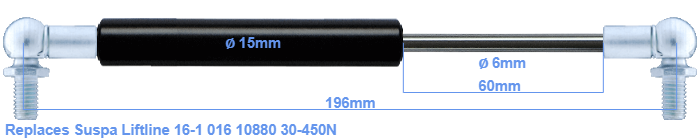# Replacement for Suspa Liftline 16-1 016 10880 30-450N

\$31.17

Replacement gas spring for the Suspa Liftline 16-1 016 10880 30-450 Newton. This fits a (possibly already present) ball with a diameter of 10mm. A corresponding ball is included. Brand: Stabilus Industry Line.
 Force Choose an option30 Newton40 Newton50 Newton60 Newton80 Newton100 Newton120 Newton140 Newton150 Newton160 Newton180 Newton200 Newton220 Newton240 Newton250 Newton260 Newton280 Newton300 Newton320 Newton340 Newton350 Newton360 Newton380 Newton400 Newton420 Newton440 Newton450 NewtonClear
This gas spring is also known as 16-1 016 10880A, 16-1 016 10880B.The diameter of the black cylinder of this gas spring is 15 millimeter. The diameter of the rod totals 6 mm. The rod (the chrome part) is 60 mm long. The total length of this replacement is 196 millimeter. This is the distance between the centers of the mounting parts. Without the mounting parts this gas spring is 152 mm long (thread to thread). The force of this replacement gas spring is 30-450 Newton. Attention: this is not an official Suspa Liftline gas spring, but a replacement gas spring. This a gas spring with the brand Stabilus Industry Line. Nevertheless, the force and dimensions equal.
Category: